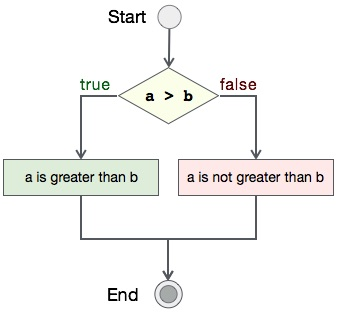# Progra to check if file exists

## Algorithm

Let's first see what should be the step-by-step procedure to compare two integers−

```START

Step 1 → Take two integer variables, say A & B

Step 2 → Assign values to variables

Step 3 → Compare variables if A is greater than B

Step 4 → If true print A is greater than B

Step 5 → If false print A is not greater than B

STOP
```

## Flow Diagram

We can draw a flow diagram for this program as given below −## Pseudocode

Let's now see the pseudocode of this algorithm −

```procedure compare(A, B)

IF A is greater than B
DISPLAY "A is greater than B"
ELSE
DISPLAY "A is not greater than B"
END IF

end procedure
```

## Implementation

Now, we shall see the actual implementation of the program −

```#include <stdio.h>
#include <sys/stat.h>

int main()
{
FILE *fp;
struct stat st;
char *filename = "sample.txt";
int flag;

flag = stat(filename, &st);

if(!flag)
printf("File exists.");
else
printf("File does not exist.");

return 0;
}
```

## Output

Output of this program should be −

```File does not exist.
```